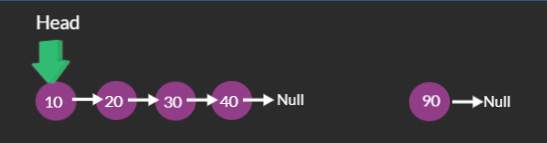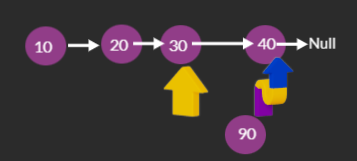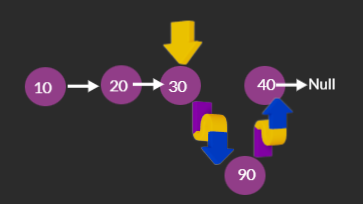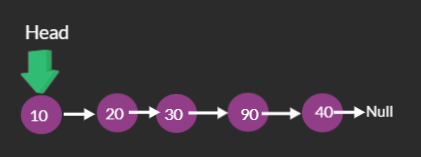# Insert at given position in Linked List

Problem Statement: Given a Singly LinkedList. Insert a Node at a given position ( 0 based Indexing ) in LinkedList.

Examples:

```Example 1:
Input: 10→20→30→40→null, value = 90, pos = 3
Output: 10→20→30→90→40→null.
Explanation: For a given LinkedList 10→20→30→40→null ,We need to insert node with value 90 at Position 3. That mean, insert the node with val 90 after the node 30.
LinkedList after inserting node 90 at position 3 becomes 10→20→30→90→40→null.

Example 2:
Input: 10→20→30→40→null, value = 100, pos = 0
Output: 100→10→20→30→40→null.
Explanation: For a given LinkedList 10→20→30→40→null ,We need to insert node with value 100 at Position 0. That mean insert the node with val 100 at beginning of LinkedList.
LinkedList after inserting node 100 at position 0 becomes 100→10→20→30→40→null.
```

### Solution :

Disclaimer: Don’t jump directly to the solution, try it out yourself first.

### Approach:

Let n be the no of Nodes in LinkedList. There are 3 cases possible for inserting a Node at a given position in Singly LinkedList.

Case 1: Insert at position 0

Case 2 : insert at position P where 0 < P <=n

Case 3 : insert at position P where P > n

In Case 1 Node is to be inserted at position 0, Which means, it is nothing but inserting the Node at beginning of LinkedList.  Inserting Node at beginning of LinkedList is 3 step process.

• Create a new node with a given value.
• Point the next newly created node to head.
• As the Node, is inserted at the beginning, this is the first node, make head point to Newly Inserted node

In Case 2 Node is to be inserted at position P where 0 < P <=n

Inserting a Node at position P in Singly LinkedList is 4 step process.

• Create a new Node with a given value.• We need to insert the new Node at position P. To do that First we should reach Position P-1. Create ListNode pointer curr, which initially points to head. Traverse the LinkedList Until the curr reaches Node which is at position P-1.• Now the curr points to the Node which is at positon P-1. We need to insert a Node at position P, which is nothing but inserting node after the curr Node. So make the next of Node which is to be inserted point to curr->next. This make sures that the, all the nodes after curr Node will now, come after the inserted Node.• Now make curr->next point to node to be inserted. This make sures that the new node is inserted at Positon PFinal LinkedList After inserting Node at Given positionIn Case 3 Node is to be inserted at position P which is greater than n. There are only n Nodes in the LinkedList. The node can be inserted at Position K where 0<=K<=n. Position P > n is invalid positon. The node cannot be inserted at such positions.

Code:

## C++ Code

``````#include <bits/stdc++.h>

using namespace std;

class ListNode {
public:
int val;
ListNode * next;
ListNode(int x) {
val = x;
next = nullptr;
}
};

ListNode * head, * tail; // head and tail of the LinkedList

void PrintList() // Function to print the LinkedList
{
ListNode * curr = head;
for (; curr != nullptr; curr = curr -> next)
cout << curr -> val << "-->";
cout << "null" << endl;
}

void InsertatLast(int value) // Function for creating a LinkedList
{

ListNode * newnode = new ListNode(value);
if (head == nullptr)
head = newnode, tail = newnode;
else
tail = tail -> next = newnode;
}

int Length() {
int len = 0;
for (ListNode * curr = head; curr != nullptr; curr = curr -> next)
len++;
return len;
}

void InsertatPositon(int value, int pos) {

ListNode * newnode = new ListNode(value);

int len = Length();

if (pos <= len) {
if (pos == 0) {
newnode -> next = head;
} else {
ListNode * curr = head;
while (--pos)
curr = curr -> next;
newnode -> next = curr -> next;
curr -> next = newnode;
}
} else
cout << "Invalid Positon" << endl;
}

int main() {
InsertatLast(10);
InsertatLast(20);
InsertatLast(30);
InsertatLast(40);
cout << "Intial LinkedList : " << endl;
PrintList();
InsertatPositon(90, 3);
cout << "LinkedList after Inserting node, with value 90, at position 3 : "
<< endl;
PrintList();
InsertatPositon(100, 0);
cout << "LinkedList after Inserting node, with value 100, at position 0 : "
<< endl;
PrintList();
return 0;
}``````

.Output:

10–>20–>30–>40–>null
LinkedList after Inserting node, with value 90, at position 3 :
10–>20–>30–>90–>40–>null
LinkedList after Inserting node, with value 100, at position 0 :
100–>10–>20–>30–>90–>40–>null

Time Complexity: O(N) Because, We are traversing the LinkedList, to find out the node at Position P-1 in LinkedList. N is the No of Nodes in LinkedList.

Space Complexity: O(1) We are not using any extra space.

## Java Code

``````import java.util.*;

class ListNode {

int val;
ListNode next;
ListNode(int x) {
val = x;
next = null;
}
}

class TUF {

static ListNode head, tail; // head and tail of the LinkedList

static void PrintList() // Function to print the LinkedList
{
ListNode curr = head;
for (; curr != null; curr = curr.next)
System.out.print(curr.val + "-->");
System.out.println("null");
}

static void InsertatLast(int value) // Function for creating a LinkedList
{

ListNode newnode = new ListNode(value);
if (head == null) {
tail = newnode;
} else
tail = tail.next = newnode;
}

static int Length() {
int len = 0;
for (ListNode curr = head; curr != null; curr = curr.next)
len++;
return len;
}

static void InsertatPositon(int value, int pos) {

ListNode newnode = new ListNode(value);

int len = Length();

if (pos <= len) {
if (pos == 0) {
} else {
ListNode curr = head;
while (--pos != 0)
curr = curr.next;
newnode.next = curr.next;
curr.next = newnode;
}
} else
System.out.println("Invalid Positon");
}

public static void main(String args[]) {
InsertatLast(10);
InsertatLast(20);
InsertatLast(30);
InsertatLast(40);
System.out.println("Intial LinkedList : ");
PrintList();
InsertatPositon(90, 3);
System.out.println("LinkedList after Inserting node, with value 90,
at position 3 : ");
PrintList();
InsertatPositon(100, 0);
System.out.println("LinkedList after Inserting node, with value 100,
at position 0 : ");
PrintList();
}
}``````

.Output: